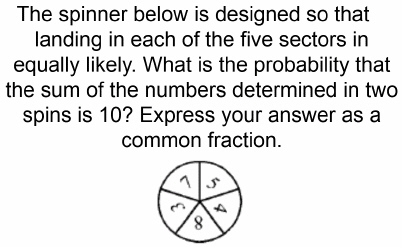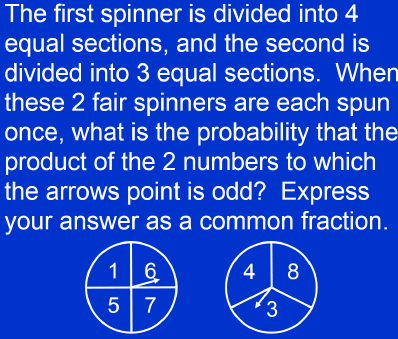Name:    Probability Questions

 Numeric Response 1. Three marbles are randomly selected, without replacement, from a bag containing two red, two blue and two green marbles. What is the probability that one marble of each color is selected? Express your answer as common fraction. 2.3. Each of six softball teams is to play each other team once. How many games are needed? 4. What is the probability that a randomly-selected two-digit number is a multiple of 14? Express you answer as common fraction 5. Sixty percent of all cereal boxes have a prize inside.  What is the probability that two randomly selected cereal boxes both contain a prize?  Express your answer as a common fraction 6. A six-faced die is weighted so that the probabilities of rolling a 1, 2 and 3 are equally likely; the probabilities of rolling a 4, 5 and 6 are equally likely; and the probability of rolling a 5 is four times the probability of rolling a 3.  What is the probability that a 2 or a 5 occurs on a single roll of the die?  Express your answer as a common fraction 7.8. Joshua flips three coins.  What is the probability that they are neither all heads nor all tails?  Express your answer as a common fraction. 9. In how many ways can a pair of students be selected from a group of five students? 10. Melinda will roll two standard six-sided dice and make a two-digit number with the two numbers she rolls.  For example, if she rolls a 6 and 3, she can either form 36 or 63.  What is the probability that she will be able to make an integer between 10 and 20?  Express your answer as a common fraction 11. A bag contains 2 red chips and 3 blue chips. Kay will take two chips out of the bag at the same time. What is the probability that the chips will be different colors? Express your answer as a common fraction 12. A team averages 7 losses for every 13 games that it wins.  If ties are not permitted, what is the probability that the team will win its next game?  Express your answer as a percent. 13. When three fair 6-sided dice are tossed, what is the probability of tossing at least one 6?  Express your answer as a common fraction. 14. Each of four students hands in a homework paper.  Later the teacher hands back the graded papers randomly, one to each of the students.  In how many ways can the papers be handed back such that every student receives someone else’s paper?  The order in which the students receive their papers is irrelevant. 15.Common fraction. 16. The three-digit positive integer N has a ones digit of 3.  What is the probability that N is divisible by 3?  Express your answer as a common fraction 17. An integer is randomly selected from the integers 0 through 99, inclusive. What is the probability that the integer is in the range 10 through 20, inclusive? Express your answer as a common fraction 18. Using the letters A, B, C and D it is possible to write 64 “words” of three letters. Three of these words are ABB, BAD and CCC. When the 64 words are placed in alphabetical order, what is the 19th word? 19. Owen has exactly a 50% chance of throwing a winner on each turn in ring toss. If he took three turns in ring toss and at least one of the turns was a winner, what was the probability that all three tosses were winners? Express your answer as a common fraction. 20. The Corner Deli has four types of bread, five types of meat, and three types of cheese. Sandwiches come in three kinds: one bread and one meat; one bread and one cheese; or one bread with one meat and one cheese. How many sandwich combinations are possible at The Corner Deli? 21. A four-digit positive integer (leading digit not 0) is chosen at random. What is the probability that at least two digits are the same? Express your answer as a common fraction 22. A standard six-faced die and a standard four-faced die are both rolled once. The cube’s faces are numbered 1- 6, and the tetrahedron’s faces are numbered 1- 4. What is the probability that the sum of the downward-facing faces is greater than 6?  Express your answer as a common fraction.